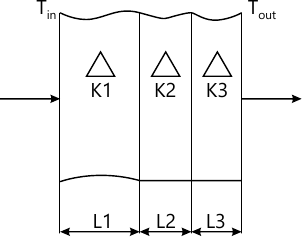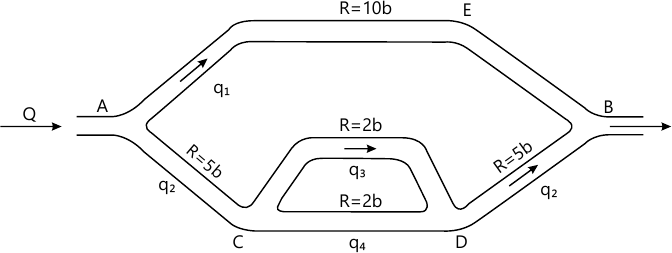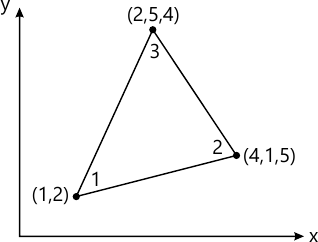MORE IN Finite Element Analysis
MU Mechanical Engineering (Semester 8)
Finite Element Analysis
December 2015
Total marks: --
Total time: --
INSTRUCTIONS
(1) Assume appropriate data and state your reasons
(2) Marks are given to the right of every question
(3) Draw neat diagrams wherever necessary

1 (a) Derive Lagrange's linear shape functions. What are the characteristics of shape functions? State the difference between shape functions and interpolation functions also plot the shape functions along length of the element.
10 M
1 (b) Explain followings:
i) Compatibility
ii) Band width
iii) Convergence criteria
iv) Node numbering scheme
v) Aspect ratio.
10 M

2 (a) Using Newton Cote's formula find values of M11 and M12. Where $$M = \int^{he}_0 x^2 \phi 1\phi_1 dx$$. $$\phi 1 = [1- (x/h_c)] \text{ and } \phi_2=(x/h_c)$$ Compare your answer with exact.
10 M
2 (b) Solve the following governing differential equation. $\dfrac{d^2y}{dx^2}- 10 x^2 = 5 \ \ BCs: 0\le x \le 1; \ y(0)= y(1)=0$ using-
i) Galerkin method with cubic polynomial for approximate function
ii) Rayleigh Ritz method mapped over entire domain using one parameter.
10 M

3 (a) Following data is given for one-dimensional, steady state, conduction heat transfer through a composite wall: Global P.V.s. Tin=800 °C. Tout=300 °C, K1=10 W/m °C, K2=50 W/m °C, K3=5 W/m °C, L1=0.10m, L2=0.20 m, L3=0.05m. Find the upknowns.10 M
3 (b) Derive shape functions for nine noded quadrilateral elements in natural coordinates.
10 M

4 (a) Analyse following fluid network completely using EME directly. Where Q, b are constants, R=branch resistance constant ΔP=Rq, ΔP = pressure drop. Establish Equation for steady state pressure and flow distribution.10 M
4 (b) Find natural frequency of axial vibration of a bar of uniform cross section of 30 mm2 and length 2m. Take, E=2×105 N/mm2 and ρ=8000 kg/m3. Take two linear elements.
10 M

5 (a) Analyse following problem completely. Assume, E=200 Gpa.10 M
5 (b) Calculate the linear interpolation functions for the linear triangular element shown in figure below -10 M

6 (a) Define:
i) Primary variables
ii) Element
iii) Nodes
iv) Natural coordinates
v) Convergence
5 M
6 (b) Develop element matrix equation for the most general using Rayleigh Ritz method. $$\dfrac {d}{dx} \left ( AE \dfrac {du}{dx} \right )+ f=0; \ 0\le x \le 1$$ Where, AE and f are constants. Use Lagrange's linear shape function to derive EME and use following data to solve global matrix equation.
Take 3 linear elements, A=0.2 m2 for each element, E=100GPa for each element.
At x=0, u=0
At x=L=12 cms, P=external force = 10 KN
f=0
Find displacements at nodes and the reactions.
15 M

7 (a) Solve for complete analysis. Where E=2×107 N/cm2 ρ=75×10-3 N/cm2.10 M
7 (b) Explain in short:
i) Serendipity approach
ii) Jacobian matrix
iii) Consistent and lumped mass matrices
iv) Boundary conditions
v) Iso-parametric elements.
10 M

More question papers from Finite Element Analysis